Courses

# SSC CPO Mock Test (GI & Reas) 8

## 50 Questions MCQ Test SSC CPO & Constable - Mock Tests & Previous Year Papers | SSC CPO Mock Test (GI & Reas) 8

Description
This mock test of SSC CPO Mock Test (GI & Reas) 8 for SSC helps you for every SSC entrance exam. This contains 50 Multiple Choice Questions for SSC SSC CPO Mock Test (GI & Reas) 8 (mcq) to study with solutions a complete question bank. The solved questions answers in this SSC CPO Mock Test (GI & Reas) 8 quiz give you a good mix of easy questions and tough questions. SSC students definitely take this SSC CPO Mock Test (GI & Reas) 8 exercise for a better result in the exam. You can find other SSC CPO Mock Test (GI & Reas) 8 extra questions, long questions & short questions for SSC on EduRev as well by searching above.
QUESTION: 1

Solution:
QUESTION: 2

Solution:
QUESTION: 3

### Find out which word or name will be in the middle after the words or names are arranged in the alphabetical order.

Solution:
QUESTION: 4
Choose the word that does NOT match with the two given words.
Obtuse : Unintelligent
Solution:
QUESTION: 5
Choose the group of letters which is different from others.
Solution:
QUESTION: 6
Choose the word that does NOT match with the two given words.
Laconic, Succinct
Solution:
QUESTION: 7
Complete the analogous pair.
Premium : Profit :: Discount : ?
Solution:
QUESTION: 8
Complete the analogous pair.
Happiness : Sorrow : : Comfort :?
Solution:
QUESTION: 9
Complete the analogous pair.
Export : Earning :: Import : ?
Solution:
QUESTION: 10
Complete the analogous pair.
Boat : Oar : : Bicycle : ?
Solution:
QUESTION: 11
Find the number of triangles in the given figure.
Solution:
QUESTION: 12

In the following alphabet series, how many times three letters L, S and W appear together in such a way that W comes in the middle and L and S get arranged on either sides?
M L W S A L S W N B Q W S L W P L S N O L W T R W S L

Solution:
QUESTION: 13
Group the following figures into three classes on the basis of identical properties.
Solution: 1,7,9 contain two similar elements one inside the other but not touching each other.
2,3,6 contain two similar elements one inside the other and both touching each ohter.
4,5,8 are divided into equal parts by straight lines emerging from the centre.
QUESTION: 14
Find the missing character.
Solution: In fig. (A), (915-364)=551.
In fig. (B), (789-543)=246.
$4$In fig. (C), missing number = (863-241)=622.
QUESTION: 15
Mohan goes 7 km towards South-East from his house, then he goes 14 km turning to West. After this he goes 7 km towards North West and in the end he goes 9 km towards East. How far is he from his house?
Solution:
QUESTION: 16
A child crawls 20 feet towards North, turns right and crawls 30 feet, turns right again and crawls 35 feet. He turns left now and crawls 15 feet. He turns left again and crawls 15 feet. Finally he turns to his left to crawl another 15 feet. How far is he from his starting point and in which direction?
Solution:
QUESTION: 17
Find the missing character.
Solution: The numbers in the right half form the series : 2, 3, 4, 5.
The numbers in the left half form the series : 5, 7, 9, 11.
QUESTION: 18
Find the missing character.
Solution: 165 + 4 = 169 = (13)2
7 + 2 = 9 = (3)2
14 + 11 = 25 = (X)2 = (5)2
QUESTION: 19

Choose the odd one out.

Solution:
QUESTION: 20

Choose the odd one out.

Solution:
QUESTION: 21

In the English alphabet 'BDG' is to 'CFJ' in the same way as 'EGJ' is to ______

Solution: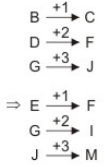QUESTION: 22
Given interchanges : signs + and - numbers 8 and 10.
Which statement is true?
Solution: Option. A
8 - 10 + 6 = 12
if we interchange the no 8 and 10 and signs + and - we get
10 + 8 - 6 = 12
⇒ 18 - 6 = 12 (correct)
Option. B
10 - 8 + 7 = 4
if we interchange the no 8 and 10 and signs + and - we get
⇒ 8 + 10 - 7 = 4
⇒ 18 - 7 = 4
⇒ 11 ≠ 4 (not true)
Option. C
10 + 8 - 9 = 16
if we interchange the no 8 and 10 and signs + and - we get
8 - 10 + 9 = 16
⇒ 17 - 10 = 16
⇒ 7 ≠ 16 (not true)
Option. D
10 + 8 - 3 = 10
if we interchange the no 8 and 10 and signs + and - we get
8 - 10 + 3 = 10
⇒ 11 - 10 = 10
⇒ 1 ≠ 10 (not true)
QUESTION: 23

In questions below, equations have become wrong due to wrong orders of signs. Choose the correct order of signs from the alternative given.

56×6÷3=29-2

Solution:
QUESTION: 24

How many such pairs of letters are there in the word SPLENIC each of which has as many letters between them in the word as there are between them in the English alphabet?

Solution: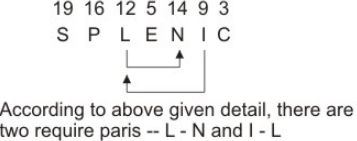QUESTION: 25

Select the correct set of symbols which will fit in the given equation 5 0 3 5 = 20.​

Solution:

5 - 0 + 3 × 5
= 5 + 15 = 20

QUESTION: 26
Complete the analogous pair.
25:37::49: ?
Solution: 25 : 37
⇒ 52 : 62 + 1
and 49 : 65
⇒ 72 : 82 + 1
QUESTION: 27

Choose the odd one out.

Solution:
QUESTION: 28

Choose the odd one out.

Solution:

Because all other numbers given in the options are prime number and 63 is a composite number

QUESTION: 29

Choose the odd one out.

Solution:
QUESTION: 30
Find out from amongst the four alternatives as to how the pattern would appear when the transparent sheet is folded at the dotted line.
Solution:
QUESTION: 31
Find out the term in the number series which is wrong.
5, 10, 17, 24, 37
Solution:
QUESTION: 32

Find the wrong number in the series.
16, 4, 2, 1.5, 1.75, 1.875,

Solution:

The series is ×0.25,×0.5,×0.75,×1,.......

QUESTION: 33
Choose the correct alternative that will continue the same pattern and fill in the blank.
1, 2, 3, 5, 8, (....)
Solution:
QUESTION: 34
3, 10, 29, (66), (127), 218
In the above number series TERMS have been put within bracket. Mark your answer as -
Solution:
QUESTION: 35
Find out the term in the number series which is wrong.
1,5,11,19,29,55
Solution: 5 - 1 = 4; 11 - 5 = 6; 19 - 11 = 8
29 - 19 = 10; 55 - 29 = 26
Pattern gets disturbed at last stage
∴ 55 is wrong
It should be 29 + 12 = 41
QUESTION: 36
Choose the missing term out of the given alternatives.
b e d f ? h j ? l
Solution:
QUESTION: 37
Below is given statement followed by two assumptions numbered I and II. You have to consider the statement and the following assumptions and decide which of the assumptions is implicit in the statement :
Statement : "If you have obtained 75 per cent or more marks in X Std examination, your admission to our coaching class for XII Std is guaranteed."-An advertisement
Assumptions:
I. Bright students do not generally opt for attending coaching classes.
II. The coaching class has adequate capacityto accomodate all such students.
Solution:
QUESTION: 38
Below is two statements followed by three conclusions numbered I, II, and III. You have to consider the statement and the following conclusions and decide which of the conclusions is follows in the statement :
Statement:
a.All roads are trees.
b. No tree is soap.
Conclusion:
I. No soap is road.
II. Some trees are roads.
III. No road is soap.
Solution:
QUESTION: 39
If Pen is coded as Pencil, Pencil as Chalk, Chalk as Slate, Slate as Paper, then by which do you mostly write?
Solution:
QUESTION: 40
Ram remembers that his brother Deepak's birthday falls after 20th May but before 28th May, while Geeta remembers that Deepak's birthday falls before 22nd May but after 12th may. On what date Deepak's birthday falls?
Solution: According to Ram ,Deepak`s birthday falls on onev of the day among 21st, 22nd, 23rd, 24th, 25th, 26th, and 27th May. According to Geeta, Deepak`s birthday falls on one of the days among 13th, 14th, 15th, 16th, 17th, 18th, 19th, 20th, and 21st May. The day common to both the groups is 21st May. .'. Deepak`s birthday falls in 21st May.
QUESTION: 41
Choose the word which is least like the other words in the group.
Solution:
QUESTION: 42
Choose one word which cannot be made from the letters of the given word
VARIEGATED
Solution:
QUESTION: 43

In the following questions, select a figure from amongst the four alternatives, which when placed in the blank space of fig. (X) would complete the pattern.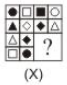Solution:
QUESTION: 44

In each question below is given a group of letters followed by four combinations of digits numbered (A), (B), (C) and (D). You have to find put which of the combinations correctly represents the group of letters based on the following coding system and mark the number of that combination as the answer. If none of the four combinations correctly represents the group of letters, mark (E) i.e. 'None of these' as the answer.
Letter/Symbol : M = S @ P  Δ D V *
Digits :                3  9 6  2  8  7 5  4  1
Conditions :
(i) If the first digit is odd and last digit is even, the codes for the first and the last digits are to be interchanged.
(ii) If the first as well as the last digit is even, both are to be coded by the code for last digit.
(iii) If the first as well as the last digit is odd, both are to be coded as 'X'.

285961

Solution:

2 → @, 8 → P, 5 → D,
9 → =, 6 → S, 1 → *

QUESTION: 45

In each question below is given a group of letters followed by four combinations of digits numbered (A), (B), (C) and (D). You have to find put which of the combinations correctly represents the group of letters based on the following coding system and mark the number of that combination as the answer. If none of the four combinations correctly represents the group of letters, mark (E) i.e. 'None of these' as the answer.
Letter/Symbol : M = S @ P  Δ D V *
Digits :                3  9 6  2  8  7 5  4  1
Conditions :
(i) If the first digit is odd and last digit is even, the codes for the first and the last digits are to be interchanged.
(ii) If the first as well as the last digit is even, both are to be coded by the code for last digit.
(iii) If the first as well as the last digit is odd, both are to be coded as 'X'.

972486

Solution:

Condition - (ii)
9 → S, 7 → Δ, 2 → @,
4 → V, 8 → P, 6 → =

QUESTION: 46

Study the following diagram.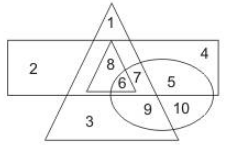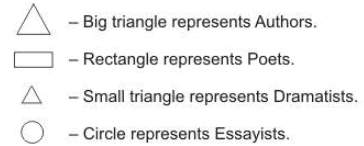Q. Which number represents poets who are also Essayists, Dramatists and Authors?

Solution:

(C) The required number should be common to all the four geometrical figures. Such number is '6'.

QUESTION: 47

Study the following diagram.Q. Which number represents Dramatists who are not Essaysists?

Solution:

(A) The number should be present in the small triangle but ouside the circle. Such number is '8'.

QUESTION: 48

Select the correct mirror image of the figure (X) from amongst the given alternatives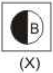Solution:
QUESTION: 49

In the following questions consists of five figures marked 1, 2, 3, 4 and 5 called the Problem Figures followed by five other figures marked A, B, C, D and E called the Answer Figures. Select a figure from the Answer Figures which will continue the same series as established by the five Problem Figures.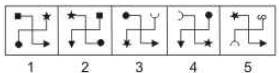Solution:
QUESTION: 50

In the questions, figure (X) is embedded in one of following figures. Find out the correct alternative.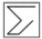Solution: